# KSEEB Solutions for Class 8 Maths Chapter 12 Construction of Triangles Additional Questions

Students can Download Maths Chapter 12 Construction of Triangles Additional Questions and Answers, Notes Pdf, KSEEB Solutions for Class 8 Maths helps you to revise the complete Karnataka State Board Syllabus and score more marks in your examinations.

## Karnataka State Syllabus Class 8 Maths Chapter 12 Construction of Triangles Additional Questions

Question 1.
Construct a triangle ABC in which AB = 5 cm, BC = 4.7 cm and AC = 4.3 cm.
Solution: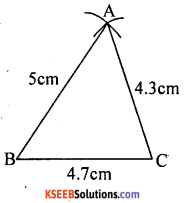Question 2.
Construct a triangle ABC in which AB = 5 cm, BC = 5 cm and AC = 4.3 cm.
Solution:Question 3.
Construct a triangle PQR in which PQ = 4 cm, QR = 4.5 cm and ∠Q = 60°.
Solution:Question 4.
Construct a triangle PQR in which PQ = 4 cm, ∠P = 60° and ∠Q = 60°.
Solution:Question 5.
Construct a triangle ABC in which AB = 3.5 cm, AC = 4 cm and length of the perpendicular from A to BC is 3 cm.
Solution:Question 6.
Construct an isosceles triangle ABC in which base BC = 4.5 cm and altitude from A on BC is 3.8 cm.
Solution:Question 7.
Construct an isosceles triangle whose altitude is 5 cm and whose vertex angle is 70°.
Solution: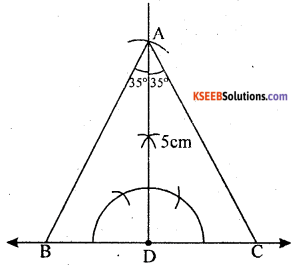Question 8.
Construct an isosceles triangle whose altitude is 5 cm and whose vertex angle is 80°.
Solution: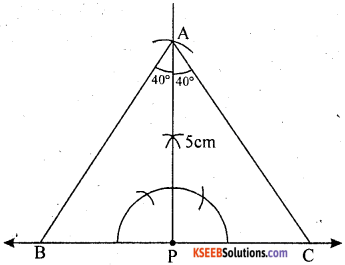Question 9.
Construct an equilateral triangle of height 3.5 cm.
Solution:Question 10.
Construct an equilateral triangle of height 4.3 cm.
Solution:Question 11.
Construct right angle triangle LMN in which M = 90°, MN = 4.5 cm and LN = 5.6cm.
Solution:Question 12.
Construct right angle triangle PQR in which Q = 9°, QR = 4.5 cm and R = 50°.
Solution: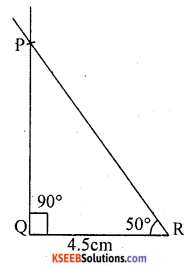Question 13.
Construct a triangle PQR, whose perimeter is 13 cm and whose sides are in the ratio 2 : 3 : 4.
Solution: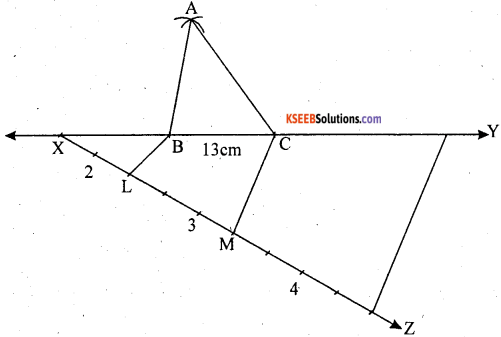Question 14.
Construct a triangle PQR, whose perimeter is 15 cm and whose sides are in the ratio 3 : 4 : 6.
Solution: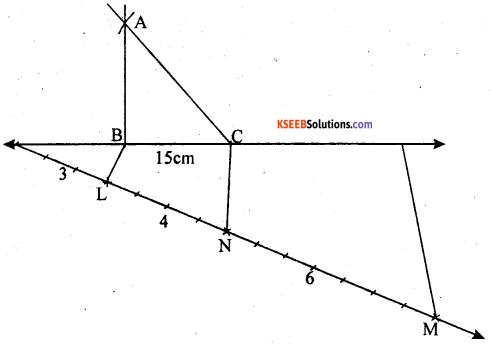Question 15.
Construct a triangle ABC, whose perimeter is 13.5 cm and whose base angles are 60° and 75°.
Solution:Question 16.
Construct a triangle ABC, whose perimeter is 12.5 cm and whose base angles are 50° and 80°.
Solution:Question 17.
Construct a triangle XYZ in which YZ = 4.5 cm, Y = 60° and sum of other two sides is 7.5 cm.
Solution: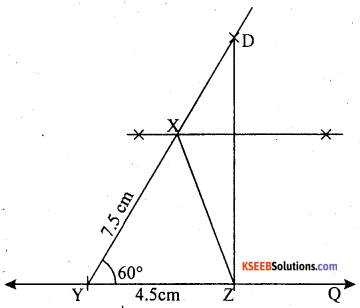Question 18.
Construct a triangle ABC whose perimeter is 9 cm and the angles are in the ratio 3 : 4 : 5.
Solution: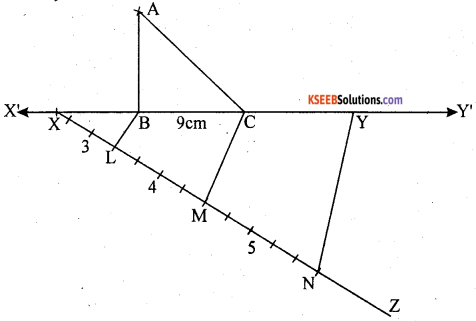Question 19.
Construct a triangle ABC whose perimeter is 12 cm and the angles are in the ratio 2 : 3 : 5.
Solution:Question 20.
Construct a triangle ABC in which BC = 4.5 cm, B = 35° and difference between the other two sides is 2.8 cm.
Solution: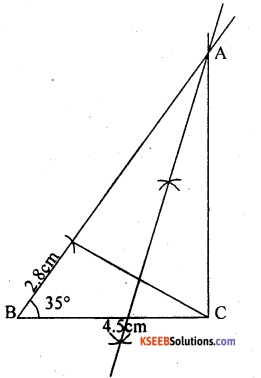error: Content is protected !!